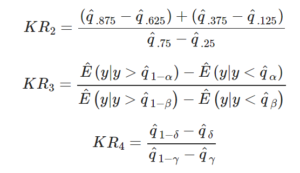Related Articles

# statsmodels.expected_robust_kurtosis() in Python

• Last Updated : 10 May, 2020

With the help of `statsmodels.expected_robust_kurtosis()` method, we can calculate the expected value of robust kurtosis measure by using `statsmodels.expected_robust_kurtosis()` method.Syntax : `statsmodels.expected_robust_kurtosis(ab, db)`

Return : Return the four kurtosis value i.e kr1, kr2, kr3 and kr4.

Example #1 :
In this example we can see that by using `statsmodels.expected_robust_kurtosis()` method, we are able to get the expected value of robust kurtosis measure by using this method.

 `# import numpy and statsmodels``import` `numpy as np``from` `statsmodels.stats.stattools ``import` `expected_robust_kurtosis``   ` `# Using statsmodels.expected_robust_kurtosis() method``gfg ``=` `expected_robust_kurtosis()``   ` `print``(gfg)`

Output :

[3.0000000 1.23309512 2.58522712 2.90584695]

Example #2 :

 `# import numpy and statsmodels``import` `numpy as np``from` `statsmodels.stats.stattools ``import` `expected_robust_kurtosis``   ` `# Using statsmodels.expected_robust_kurtosis() method``gfg ``=` `expected_robust_kurtosis([``12``, ``22``], [``6``, ``7``])``   ` `print``(gfg)`

Output :

[3.0000000 1.23309512 1.23859789 1.0535188 ]

Attention geek! Strengthen your foundations with the Python Programming Foundation Course and learn the basics.

To begin with, your interview preparations Enhance your Data Structures concepts with the Python DS Course. And to begin with your Machine Learning Journey, join the Machine Learning – Basic Level Course

My Personal Notes arrow_drop_up